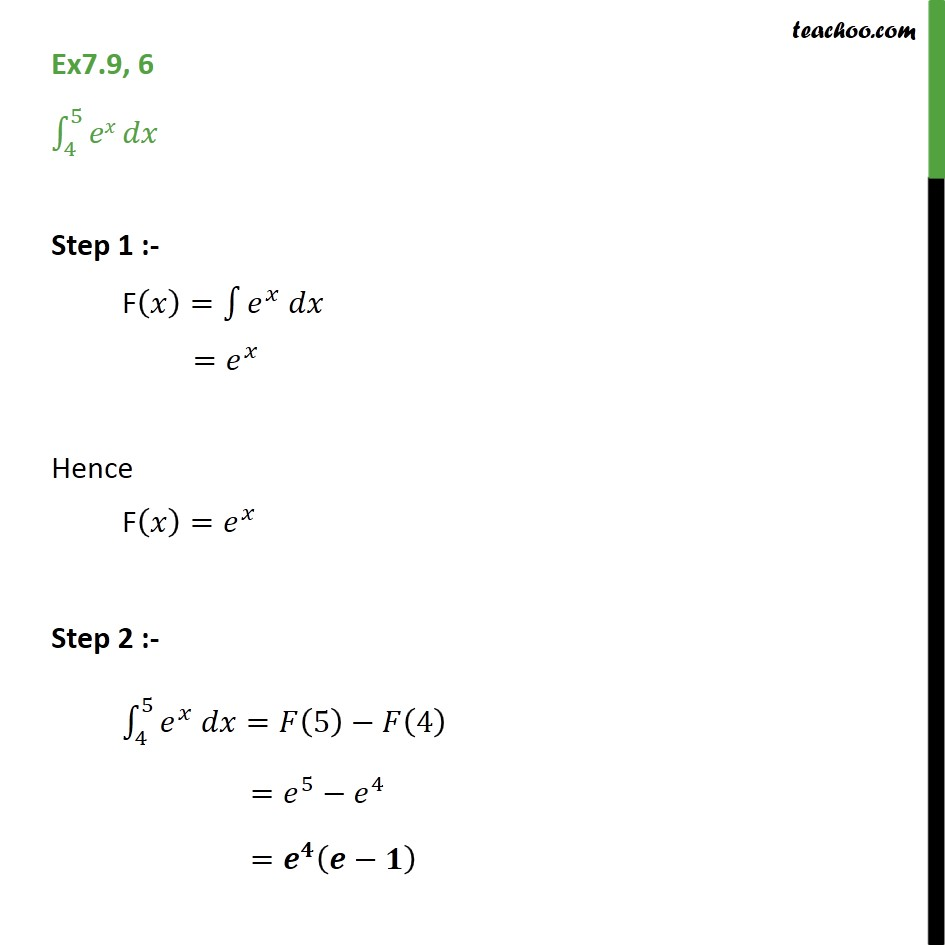1. Chapter 7 Class 12 Integrals (Term 2)
2. Serial order wise
3. Ex 7.9

Transcript

Ex 7.9, 6 - Chapter 7 Class 12 Integration - Teachoo Direct Integrate ∫ 4 -> 5 e^x dx Step 1 :- F(x) = ∫ e^x dx = e^x Hence F(x) = e^x Step 2 :- ∫ 4 -> 5 e^x dx = F(5) - F(4) = e^5 - e^4 = e^4 (e - 1)

Ex 7.9